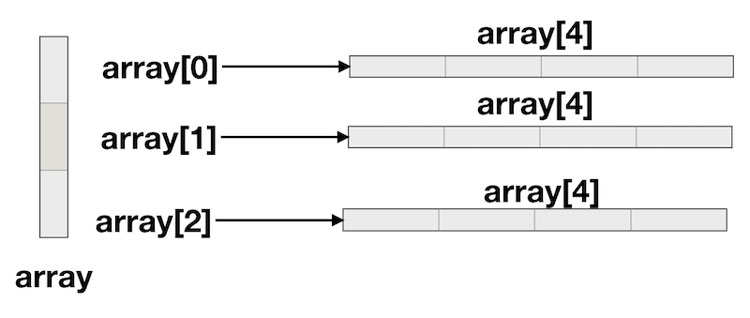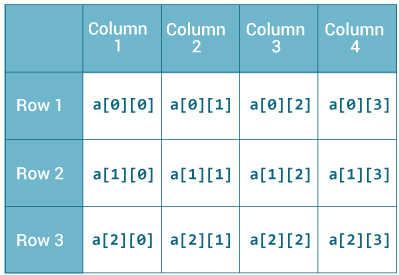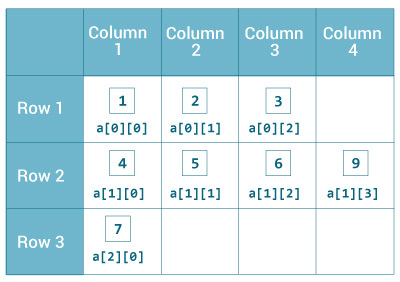# blog posts# In Java, you can define an array of arrays known as multidimensional arrays. Before learning about multidimensional arrays.

In that tutorial, you learned how to create and use an array of primary data types (such as Double, int, etc.), a string array, and an object array. An array of arrays can also be created. For example,

int [] [] a = new int  ;

#### Here a is a two-dimensional array that holds up to 12 integer elements.Note that the array starts from 0, not 1.

You can also define a three-dimensional array. for example:

String [] [] [] personalInfo = new String   ;

Here personalInfo is a 3D array that holds up to 24 string elements.

In Java, the components of a multidimensional array are also arrays.

If you are familiar with C ++ / C, you may feel that multidimensional arrays work similarly in Java and C ++ / C. Well, it is not. In Java, rows can have different lengths.

You will notice the difference when initializing.

## How to initialize a 2D array in Java?

Here is an example of how to initialize a 2D array in Java.

int [] [] a = {

{1, 2, 3},

{4, 5, 6, 9},

{7},

};

As mentioned, each component of array a is an array in itself, and the length of each row is different.#### Let’s write a program to prove it.

1. class MultidimensionalArray {
2. public static void main (String [] args) {
3. int [] [] a = {
4. {1, 2, 3},
5. {4, 5, 6, 9},
6. {7},
7. };
8. System.out.println (“Length of row 1:” + a  .length);
9. System.out.println (“Length of row 2:” + a  .length);
10. System.out.println (“Length of row 3:” + a  .length);
11. }
12. }

#### Output

Length of row 1: 3

Length of row 2: 4

Length of row 3: 1

Since each component of a multidimensional array is also an array ([a , a [1, and [a [2 are also arrays)), you can use the length property to find the length of each row.

#### Example: Print all the elements of a 2D array using a loop

1. class MultidimensionalArray {
2. public static void main (String [] args) {
3. int [] [] a = {
4. {1, -2, 3},
5. {-4, -5, 6, 9},
6. {7},
7. };
8. for (int i = 0; i <a.length; ++ i) {
9. for (int j = 0; j <a [i] .length; ++ j) {
10. System.out.println (a [i] [j]);
11. }
12. }
13. }
14. }

#### Output

It may be best to use the for… each loop at all times. You can do the same with the for… each loop:

1. class MultidimensionalArray {
2. public static void main (String [] args) {
3. int [] [] a = {
4. {1, -2, 3},
5. {-4, -5, 6, 9},
6. {7},
7. };
8. for (int [] innerArray: a))
9. for (int data: innerArray) {
10. System.out.println (data);
11. }
12. }
13. }
14. }

1

-2

3

-4

-5

6

9

7

#### How to initialize a 3D array in Java?

You can set the 3D array in the same way as a 2D array. Consider the following example:

// test is a 3d array

int [] [] [] test = =

{

{1, -2, 3},

{2, 3, 4}

},

{

{-4, -5, 6, 9},

{1},

{2, 3}

}

};

In essence, a three-dimensional array is an array of two-dimensional arrays.

Similar to 2D arrays, rows of 3D arrays can have different lengths.

#### Example: A program for printing 3D array elements using loops

1. class ThreeArray {
2. public static void main (String [] args) {
3. // test is a 3d array
4. int [] [] [] test = =
5. {
6. {1, -2, 3},
7. {2, 3, 4}
8. },
9. {
10. {-4, -5, 6, 9},
11. {1},
12. {2, 3}
13. }
14. };
15. // for..each loop to iterate through elements of 3d array
16. for (int [] [] array2D: test) {
17. for (int [] array1D: array2D) {
18. for (int item: array1D) {
19. System.out.println (item);
20. }
21. }
22. }
23. }
24. }

1

-2

3

۲

3

4

-4

-5

6

9

1

۲

3